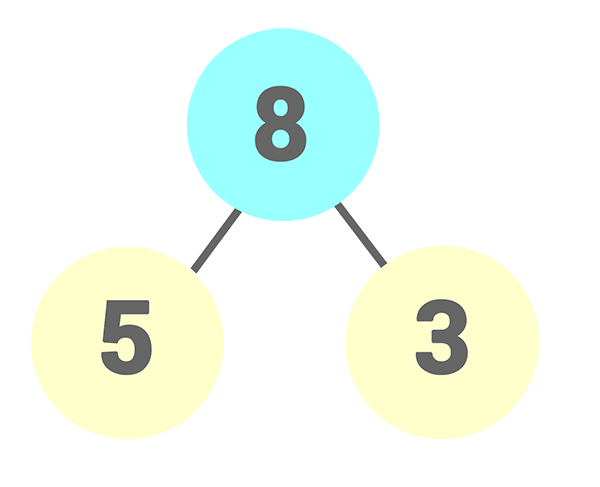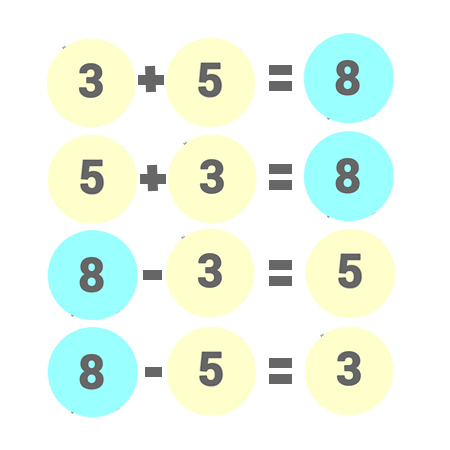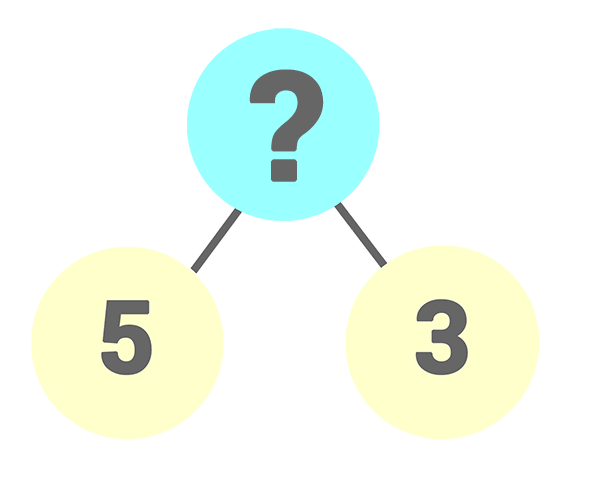# Learning “Singapore Math” through Number Bonds

The way Mathematics is taught in Singapore schools has attracted a lot of global attention in recent years. This is because students in Singapore have outperformed their peers from many developed and developing countries in the subjects of Mathematics and Science according to Trends in International Mathematics and Science Study (TIMSS). TIMSS is a widely recognised achievement test by educators and governments globally. “Singapore Math” has also started catching on in American schools where tools like counting blocks and techniques like number bonds help to play an important part in building up the students foundation.

## Singapore Math

Singapore Math curriculum focus more on the mastery of the various concepts rather than just equation solving. Students begin Primary 1 by learning basic skills and concepts, such as counting from one to 10, number bonds, addition and subtraction. Basic understanding of multiplication (in terms of repeated additions) and division concepts are also taught. All these helped to lay a good foundation for the students to pick up more advance level of the concepts later on.

In recent years, Math has been taught more visually in Singapore schools. Through the use of counting blocks and techniques like number bonds, students are able to see and learn Math with better understanding.

## Number Bonds

Number bonds has been regarded as one of the best ways to teach basic Math concepts like addition and subs-traction. A number bond diagram like the one below shows the relationship of 2 Parts (3,5) and 1 Whole (8).Students will learn to see that the two Parts can be added up in any order to form the Whole. And when one of the Part is taken away from the Whole, they will just be left with the other part.After a student understands the relationships of the 2 Parts and Whole, the concept can then be further extended to addition and subtraction as illustrate in the diagram below. Making it easier for the student to understand the concept of addition and subtraction.

Students will be practicing the few scenarios below. Once they are familiar with the number bonds, they will be able to recognize and complete it almost instantly.If you are interested to learn more about how to teach your students or kids Number Bonds, do check out our App where students can practise learning Number Bonds the fun way.